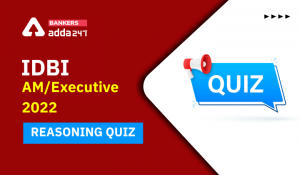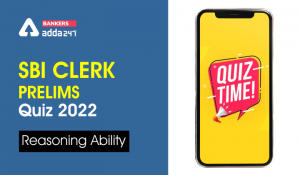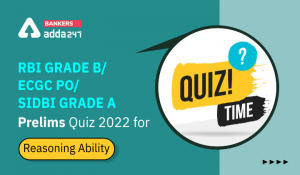Latest Banking jobs   »

# Reasoning Ability Quiz For IBPS RRB PO Prelims 2022- 3rd June

Directions (1-5): On the basis of the numbers given below, answer the following questions:

266   789   456   763   279

Reasoning Quiz For IBPS RRB PO Prelims 2022 3rd June CHECK IN HINDI

Q1. What is the difference between the two numbers which are exactly divisible by 3 but not by 6?
(a) 26
(b) 226
(c) 333
(d) 612
(e) None of these

Q2. If all the digits within the number are arranged in decreasing order, then if the number at unit place is odd, 1 is added to it. Find, which of the following is the sum obtained by adding all the digits at unit’s place of all the numbers?
(a) 33
(b) 23
(c) 20
(d) 30
(e) None of these

Q3. If we subtract 1 from even numbers (3-digit even number), then what will be the sum of the digits at unit’s place of all the numbers?
(a) 31
(b) 32
(c) 33
(d) 34
(e) None of these

Q4. If we add 3 to every even digit which is less than 7 within the number, which one of the following is the second highest number?
(a) 266
(b) 789
(c) 456
(d) 763
(e) 279

Q5. If all the digits within a number are arranged in such a way that even digits (within a number) are arranged first in increasing order and then the odd digits are arranged. Which one of the following is the third lowest number?
(a) 266
(b) 789
(c) 456
(d) 763
(e) 279

Directions (6-10): Answer the following questions based on the alphanumeric-series given below:

A @ D 7 M Q % 5 \$ F 6 K L & 4 R # N U 5

Q6. If the symbols followed by consonants interchange their positions within the group, then which element is third from the right end?
(a) U
(b) #
(c) N
(d) 5
(e) None of these

Q7. Based on the given arrangement which of the following group of elements will be next?

(a) F4K
(b) KS7
(c) RNU
(d) C4H
(e) LS1

Q8. Which of the following is second to the left of the twelfth from the right end if all the symbols are dropped?
(a) F
(b) D
(c) 7
(d) M
(e) None of these

Q9. If the numbers which are preceded by letters interchanged their positions, after that those letters were changed to the next letter according to alphabetical series, then which of the following elements will be the fourth from the left end and eleventh from the right end?
(a) F and 7
(b) 6 and D
(c) A and \$
(d) E and 6
(e) None of these

Q10. How many letters are there which are preceded by symbol and followed by number in the given arrangement?
(a) None
(b) One
(c) Two
(d) Three
(e) More than three

Directions (11-15): Answer the following questions based on the alphanumeric symbol series given below:

% A 7 O & 8 ^ 4 Y 5 * 1 9 T ! C # 2 \$ E

Q11. If the digits and symbols are interchanged, i.e., the first digit is interchanged with the first symbol, second digit with the second symbol and so on from the left end, what will be the 14th element from the right end?
(a) ^
(b) 8
(c) 4
(d) &
(e) T

Q12. What is the 7th element to the left of the element that is 9th from the right end in the series?
(a) &
(b) C
(c) 2
(d) #
(e) \$

Q13. How many digits are there which are preceded by a symbol and followed by a letter?
(a) 0
(b) 1
(c) 2
(d) 3
(e) More than 3

Q14. If all the symbols are removed then what will be the element which is 9th from the left end?
(a) 4
(b) 7
(c) 1
(d) 9
(e) None of the above

Q15. If all the digits are arranged in decreasing order from the left, how many digits will have their positions unchanged?
(a) 0
(b) 1
(c) 2
(d) 3
(e) More than 3

Solutions

S1. Ans. (e)
Sol. First, we have to find the numbers which are divisible by 3 but not by 6. The numbers are are 789 and 279.
So, difference between these numbers is 510.

S2. Ans. (c)
Sol. When all the digits within the number are arranged in decreasing order, we get:
662  987  654  763  972
If the digit at unit’s place is odd, 1 must be added to it i.e.,
662  988  654  764  972
On adding the digits at unit’s place of all the numbers, we get 20 (i.e., 2 + 8 + 4 + 4 + 2).

S3. Ans. (a)
Sol. On subtracting 1 from all even numbers, we get: 265 789 455 63 279.
On adding digit at unit’s place, we get 31 (5 + 9 + 5 + 3 + 9).

S4. Ans. (b)
Sol. On adding 3 to every even digit which is less than 7, we get:
599  789  759  793  579
Arranging the numbers, 579 < 599 < 759 < 789 < 793
Clearly, we can see that 789 is the second highest number.

S5. Ans. (c)
Sol. When all the numbers are arranged in such a way that even digits are arranged first then the odd digits are arranged in increasing order, we get: 266 879 465 637 279
On arranging the numbers, we get: 266 < 279 < 465 < 637 < 879
Clearly, 456 (465 in the required number) is the third lowest number.

S6. Ans. (b)
Sol. New Arrangement: A D @ 7 M Q % 5 F \$ 6 K L & 4 R N # U 5
So, third element from the right end = #

S7. Ans. (c)
Sol. A @ D 7 M Q % 5 \$ F 6 K L & 4 R # N U 5
So, RNU is the correct answer.

S8. Ans. (b)
Sol. New Arrangement: A D 7 M Q 5 F 6 K L 4 R N U 5
2nd to the left of the 12th from the right = 14th from the right end
So, 14th from the right end = D

S9. Ans. (d)
Sol. New Arrangement: A @ 7 E M Q % 5 \$ 6 G K L & 4 R # N 5 V
4th element from the left end = E
11th element from the right end = 6

S10. Ans. (c)
Sol. Given Series: A @ D 7 M Q % 5 \$ F 6 K L & 4 R # N U 5
D and F are two such letters which are preceded by symbol and followed by number.

S11. Ans. (c)
Sol. Given series: % A 7 O & 8 ^ 4 Y 5 * 1 9 T ! C # 2 \$ E
The symbols and digits interchanged are %-7, &-8, ^-4, *-5, !-1, #-9, \$-2
So, the new sequence is 7 A % O 8 & 4 ^ Y * 5 ! # T 1 C 9 \$ 2 E
14th element from right is 4

S12. Ans. (a)
Sol. Given series: % A 7 O & 8 ^ 4 Y 5 * 1 9 T ! C # 2 \$ E
Element that is 9th from the right is 1 and 7th to the left of 1 is &.
So, (a.) & is the answer.

S13. Ans. (b)
Sol. Given series: % A 7 O & 8 ^ 4 Y 5 * 1 9 T ! C # 2 \$ E
Only 4 is preceded by a symbol and followed by a letter. So, (b) is the answer.

S14. Ans. (d)
Sol. Given series: % A 7 O & 8 ^ 4 Y 5 * 1 9 T ! C # 2 \$ E
If the symbols are removed, the new sequence is A 7 O 8 4 Y 5 1 9 T C 2 E
So, 9 is 9th from the left end.

S15. Ans. (c)
Sol. Given series: % A 7 O & 8 ^ 4 Y 5 * 1 9 T ! C # 2 \$ E
New Sequence % A 9 O & 8 ^ 7 Y 5 * 4 2 T ! C # 1 \$ E
So, only 8 and 5 have unchanged positions.#### Congratulations!Incorrect details? Fill the form again here

•Reasoning Ability Quiz For IDBI AM/Execu...
•Reasoning Ability Quiz For IBPS RRB PO P...
•Reasoning Ability Quiz For IBPS RRB PO P...
•Reasoning Ability Quiz For SBI Clerk Pre...
•Reasoning Ability Quiz For SBI Clerk Pre...
•Reasoning Ability Quiz For RBI Grade B/ ...